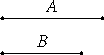# Proposition 8

If two magnitudes do not have to one another the ratio which a number has to a number, then the magnitudes are incommensurable.

Let the two magnitudes A and B not have to one another the ratio which a number has to a number.I say that the magnitudes A and B are incommensurable.

X.5

For, if they are commensurable, then A has to B the ratio which a number has to a number.

But it does not, therefore the magnitudes A and B are incommensurable.

Therefore, if two magnitudes do not have to one another the ratio which a number has to a number, then the magnitudes are incommensurable.

Q.E.D.

## Guide

This proposition is the contrapositive of X.5. It is used in frequently in X.11.